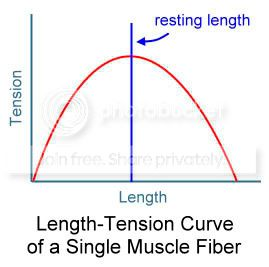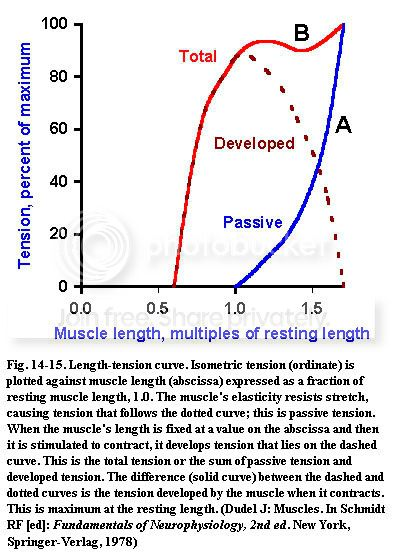# Зависимость напряжения мышцы от длины

Извиняюсь, только на английском http://www.pt.ntu.edu.tw/hmchai/BM03/BMmaterial/Muscle.htm#factor

Mechanical Properties of the Skeletal Muscle

Length-Tension Relationship
# The tension that a muscle generates varies with its length
# found when a muscle under isometric contraction and for maximum activation of the muscle
# In a single muscle fiber,
# peak force is noted at normal resting length.
# a bell-shaped length-tension curve

In a muscle, force generation capacity increases when the muscle is slightly stretched because of the effect of both active and passive components.http://www.unmc.edu/physiology/Mann/mann14.html
Length-tension relation

When a resting skeletal muscle is stretched from its resting length, i.e., its length at rest in the body, the parallel elastic elements are stretched and tension increases along the blue curve (A) in Figure 14-15. In this figure, length, expressed as a fraction of resting length, is plotted on the abscissa against tension (or force) on the ordinate. If the muscle is stretched to about 180% of its resting length (this is about the maximum stretch without damage to the muscle), and the length is held constant at this value while a contraction is induced (the set up for this experiment is shown in Figure 14-14), the maximum isometric tension of the muscle is obtained. This tension (total tension) is the sum of the parallel elastic tension (i.e., passive tension) and the contractile tension (i.e., active tension).

At any length less than 180% of the resting length, the total tension developed by the muscle during an isometric contraction will be less and will follow the red curve (B) in Figure 14-15. To compute the contractile tension, the tension developed by the contractile elements, we simply subtract the blue curve (A) from the red curve (B). The result is the solid curve, sometimes referred to as an isometric length-tension curve.Obviously, the maximum isometric contractile tension occurs when the muscle is at its resting length. At shorter or longer lengths, the isometric tension produced by the contractile elements is less. The exact shape of the curve for lengths longer than the resting length depends upon when and how the tension is measured (for a discussion see Noble MIM, Pollack GH: Molecular mechanisms of contraction. Circ Res 40:333-342, 1977).

At lengths less than about 70% of resting length, the muscle develops no tension at all when stimulated. It follows that, during an isotonic contraction, a skeletal muscle can only shorten to about 70% of its resting length, and it can only develop tension at lengths between 70% and 180% of resting length. The isotonic length-tension curve approximately superimposes upon the isometric curve; hence during an isotonic contraction the muscle shortens to a length appropriate for the tension developed (i.e., to a length predictable from the isometric length-tension curve).

The length-tension curve can be explained by the cross-bridge theory. Instead of the length of the whole muscle, we could just as accurately have plotted sarcomere length on the abscissa of Figure 14-15, with resting length equal to about 2 µm. At resting length, the thin and thick filaments are in relative positions as shown in Figure 14-7C, with nearly the whole thick filament overlapped by thin filaments. In this position, all of the myosin heads are overlapped by the thin filament, and therefore all are available to form cross-bridges. Recall that in the cross-bridge theory the force of contraction is proportional to the number of cross-bridges formed. At longer lengths, such as those in A and B of Figure 14-7, some of the myosin heads are not overlapped by actin and therefore are not available to form cross-bridges. As a consequence, the tension developed will be less. At shorter lengths, such as D, the actin filaments from opposite ends of the sarcomere begin to interfere with each other and at shortest lengths the Z disc may block or otherwise impede movement of the thick filament
Tags:

• #### Протокол против вирусов

Лекарства от модной болезни. Только он не пишет про дозировку Ивермектина: людям с положительным результатом теста по одной 12 мг таблетке в…

• #### Рапамицин (сиролимус, рапамун)

Новости года 3. Более свежей информации я не вижу. Ну, что есть. Возможно, препарат имеет смысл рассмотреть при подсадке ткани для зубных…

• Post a new comment

#### Error

default userpic